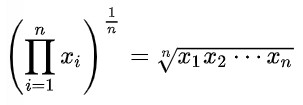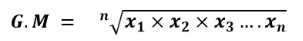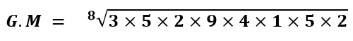# Geometric Mean Calculator

Enter the comma seprated data set values in the input box. Hit the Calculate button to find the geometric mean using geometric means calculator.

Formula:
G.M = (X1 × X2 × X2 ... × Xn) 1n

Give Us Feedback

## Geometric Mean Calculator

Geometric mean calculator is an online statistical tool that calculates the geometric mean of the sample data set.

## What is geometric mean?

Geometric mean is a type of average that indicates the central tendency of a set of numbers by using the product of their values. The geometric mean is defined as the nth root of the product of n numbers.

## Geometric mean formulaWhere,

• n is the total numbers of samples,
• x1, x2, x3,…. are individual values in the data set.

## How to find the geometric mean?

To calculate the geometric mean of the given data set, follow the below example.

Example:

Find the geometric mean of the following set of data.

3, 5, 2, 9, 4, 1, 5, 2

Solution:

Step 1: Identify the values.

x1 = 3, x2 = 5, x3 = 2, x4 = 9, x5 = 4, x6 = 1, x7 = 5, x8 = 2

N = 8

Step 2: Write the geometric mean formula and place the values.G.M = (10800)1/8

G.M = (10800)0.125

G.M = 3.1928

## Geometric Mean Table

 Geometric Mean of 4 and 9 6 Geometric Mean of 4 and 10 6.3246 Geometric Mean of 4 and 12 6.9282 Geometric Mean of 4 and 16 8 Geometric Mean of 5 and 20 10 Geometric Mean of 2 and 8 4 Geometric Mean of 2 and 25 7.0711 Geometric Mean of 8 and 12 9.798 Geometric Mean of 9 and 16 12 Geometric Mean of 8 and 18 12 Geometric Mean of 5 and 15 8.6603 Geometric Mean of 5 and 10 7.0711 Geometric Mean of 100 and 25 50 Geometric Mean of 4,6 and 9 6

### Math Tools